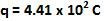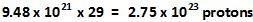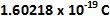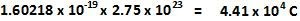## Saturday, January 16, 2010

### Irodov Problem 3.2

There are two forces acting between the copper spheres namely an attractive gravitational force and a repulsive electrostatic force. However, as we know already, the gravitational force will be several orders of magnitude weaker than the electrostatic force, hence we neglect it.

Since the molar mass of copper is 63.54 g/mol, 1 gram of copper has 1/63.54 moles of copper. The number of copper atoms in each mole of copper is given by Avogadro's number. H ence, the total number of copper atoms in 1 gram of copper is given by,Since the atomic number of copper is 29, each atom of copper has 29 protons. In other words 1 gram of copper has. The electrostatic force is this given by,Since the charge of each proton is, the total charge in all the protons in 1 gram of copper is given by,1% of the total number of protons are not compensated by the electrons, hence the total charge in each copper sphere is. The electrostatic force is given by,